# Percentile in tableauWith a quick table calculation, we can in Tableau compute percentiles for a set of values.

When to use Percentile Calculation?

When we are interested in comparing how an employee or company stands when compared to the rest of companies/ employees in the field; to be able to compare performance, we need a statistical method that reports relative standing – the percentile.

How to we use it in Tableau?

By using percentile as a ranking ‘measure’. In other words as per the following example:

• One  student is looking at four-year review scores for a group of universities, so he can decide where to apply: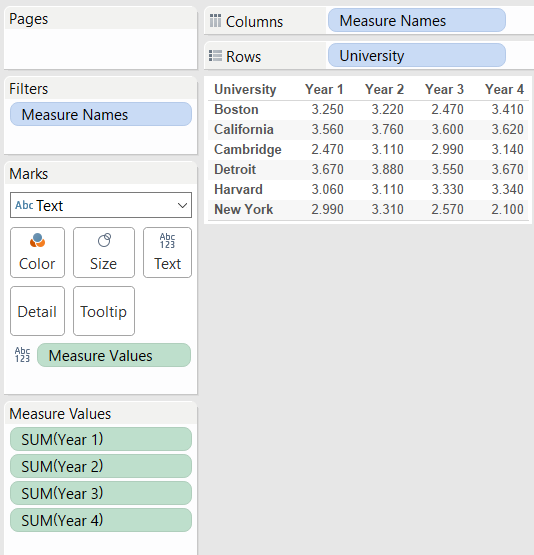The student wonders how the universities rating has changed over time, relative to each other. To find out, the student applies a Percentile table calculation to the reviews for each year, to obtain the following result: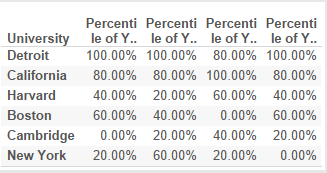The student can now observe that despite some fluctuations, the universities rating was relatively stable relative to each other during the four years.

To obtain this view, the student had to apply a Percentile table calculation to each of four measures: Year 1, Year 2, Year 3, Year 4. For each of these measures the student used tableau by:

1. Clicking the year in the view and selecting Add Table Calculation.
2. In the Table Calculation dialog box, selectedPercentile from the Calculation Type drop-down.
3. SelectedTable (Down) from the Running along
4. And chose Ascending as sorting direction.

What is then a percentile? And how do we calculate it mathematically?

The kthpercentileis a value in a data set that splits the data into two pieces: The lower section contains k percent of the data, and the upper piece contains the rest of the data (which totals to [100 – k] percent, because the total amount of data is 100%), where k is a number between 0 and 100.

Bearing in mind that the median is the 50th percentile, the ‘transition’ point where 50% of the data falls below that point, and the remaining 50% falls above it.

If we were to calculate the kth percentile (where k is any number between zero and 100), we had to:

1. Order all the values in the data set from smallest to largest.
2. Calculate an index by multiplyingk percent by the total number of values, n.
3. If the index obtained above:
• is not a whole number, we should round it up to the nearest whole number and count the values in the data set from smallest to largest value, until we reach to the number occupying the position indicated by the index;
• is a whole number, we should count the values in the data set from left to right until we reach the number occupying the position indicated by the index;

The kth percentile is then the average of that value and the value that directly follows it.

Putting the theory into practice – Let’s suppose we have 25 test scores ordered from the lowest to the highest, such as:

43, 54, 56, 61, 62, 66, 68, 69, 69, 70, 71, 72, 77, 78, 79, 85, 87, 88, 89, 93, 95, 96, 98, 99, 99

To find the 90th percentile for these (ordered) scores, we had to multiply 90% (0.9) times the total number of scores, which gives 90% ∗ 25 = 0.90 ∗ 25 = 22.5 (index calculation). Rounding it up to the nearest whole number, we then get 23.

If we now count from left to right (from the smallest to the largest value in the data set), until we find the 23rd value in the data set, that value corresponds to 98, corresponding to the 90th percentile for this data set.

Let’s say we want to find the 20th percentile:

1. Find the index, by multiplying 0.20 x 25 = 5 – this is a whole number, so the 20th percentile is the average of the 5th and 6th values in the ordered data set (62 and 66). The 20th percentile then comes to (62 + 66) ÷ 2 = 64.

For this data set the median or the 50th percentile for the test scores is the 13th score, corresponding to 77.

There are various ways of calculating percentiles, the one explained is just one of many ways. The multitude of ways to calculate percentiles may lead to approximate values and not exact values when comparing the results, don’t be alarmed is just the way of computing the percentiles.

Sours: https://www.thedataschool.co.uk/elnisa-marques/tableau-quick-calculation-calculate-percentiles

## Choosing the Right Calculation Type

The type of calculation you choose depends on the needs of your analysis, the question you want to answer, and the layout of your visualization.

### Which calculation is right for your analysis?

Choosing the type of calculation to use for your analysis is not always easy. When trying to decide, consider the following questions and examples.

Note: This content was originally published on the Tableau Blog. See A Handy Guide to Choosing the Right Calculation for Your Question(Link opens in a new window) to read it.

### Basic expression or table calculation?

##### Question 1: Do you already have all the data values you need on the visualization?

If the answer is yes: You can use a table calculation.

If the answer is no: Use a basic calculation.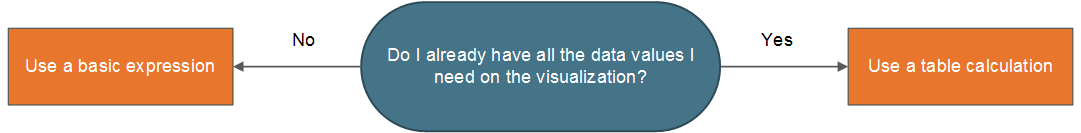##### Example:

Consider the following two visualizations. The visualization on the left is a bar chart that shows the total sales per country/region. The visualization on the right also shows sales per country/region, but sales has been disaggregated.

How could you calculate the 90th percentile of sales for each of these visualizations?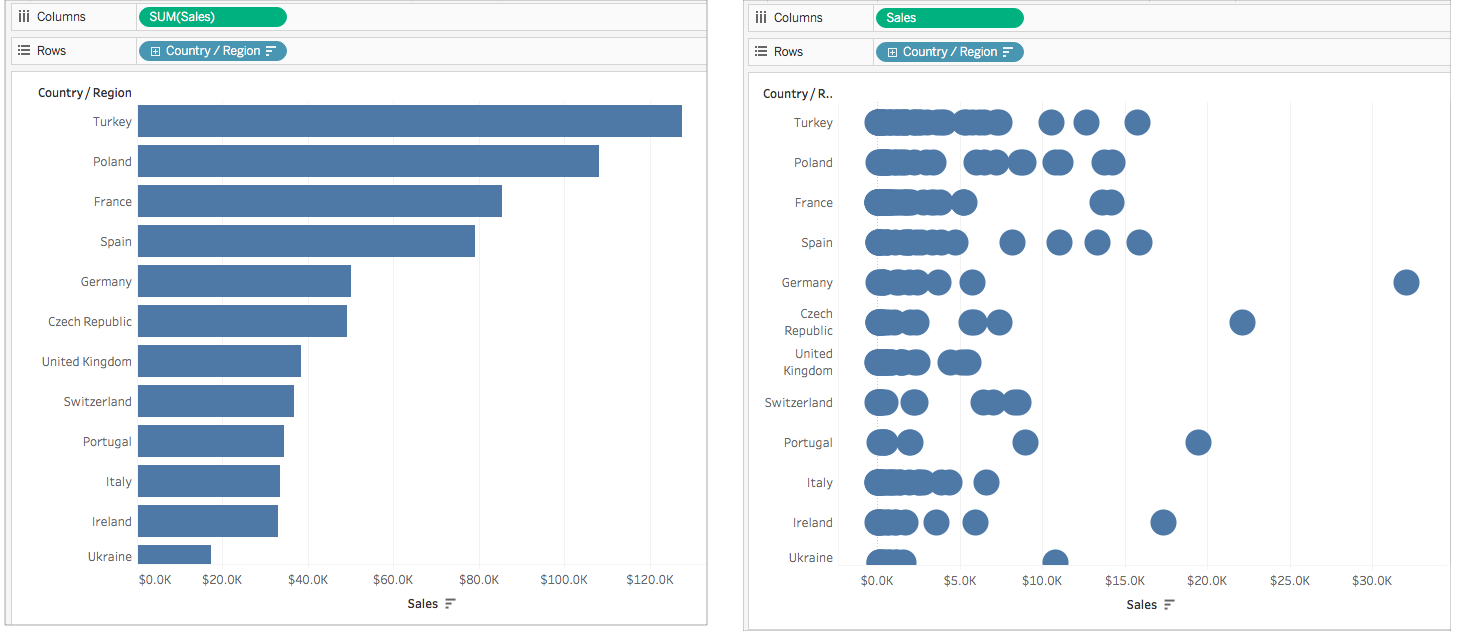The bar chart on the left is aggregated by SUM. Therefore, there is not enough detail in this view to use a table calculation. You can use a basic aggregate expression to calculate the 90th percentile of sales for each country in this example using the following formula:

This results in a value for 90th percentile per country as a label for each bar.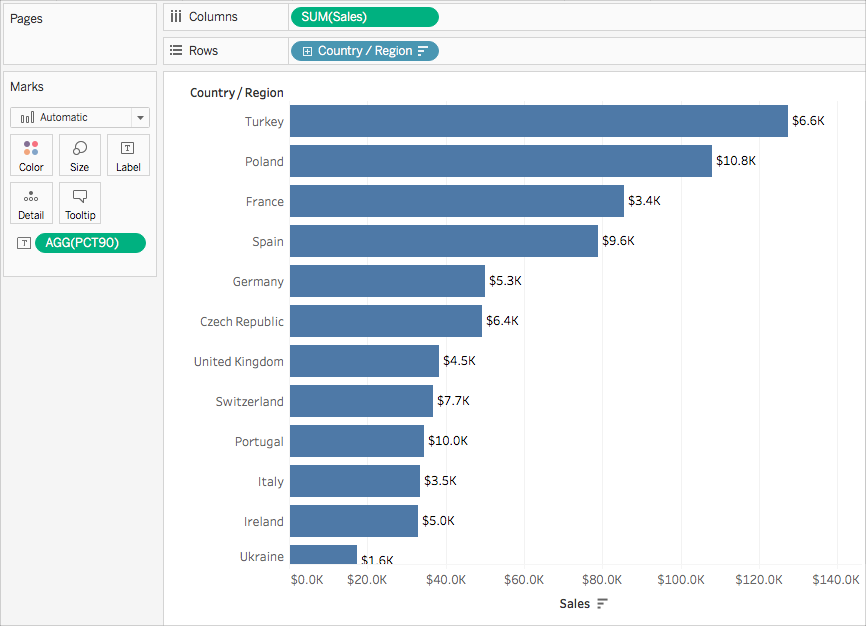However, the chart on the left includes a data value for every sales order. A larger distribution and outliers are shown. There is enough detail in the view to use a table calculation.

You can calculate the 90th percentile of sales for each country by using a distribution band (equivalent to a table calculation). There is more context in this visualization.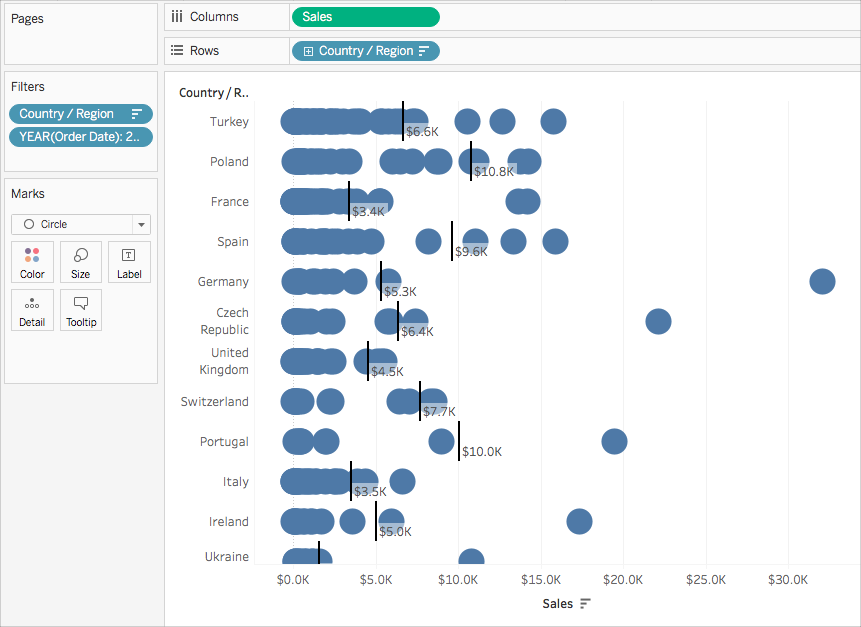Both calculations achieve the same values, but the insights you gather from each differ based on the level of detail (the amount of data) in the visualization.

### Basic expression or Level of Detail (LOD) expression?

If you don't have all the data you need on the visualization, you need your calculation to be passed through to the data source. This means you must use a basic calculation or an LOD Expression.

##### Question 2: Does the granularity of your question match either the granularity of the visualization or the granularity of the data source?

If the answer is yes: Use a basic expression.

If the answer is no: Use a Level of Detail (LOD) expression.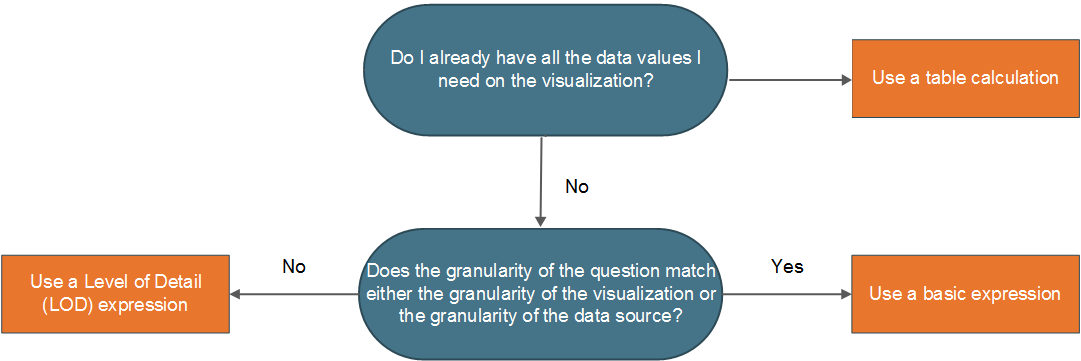##### Example

Consider the following visualization. It shows the 90th percentile of sales for all orders in each country.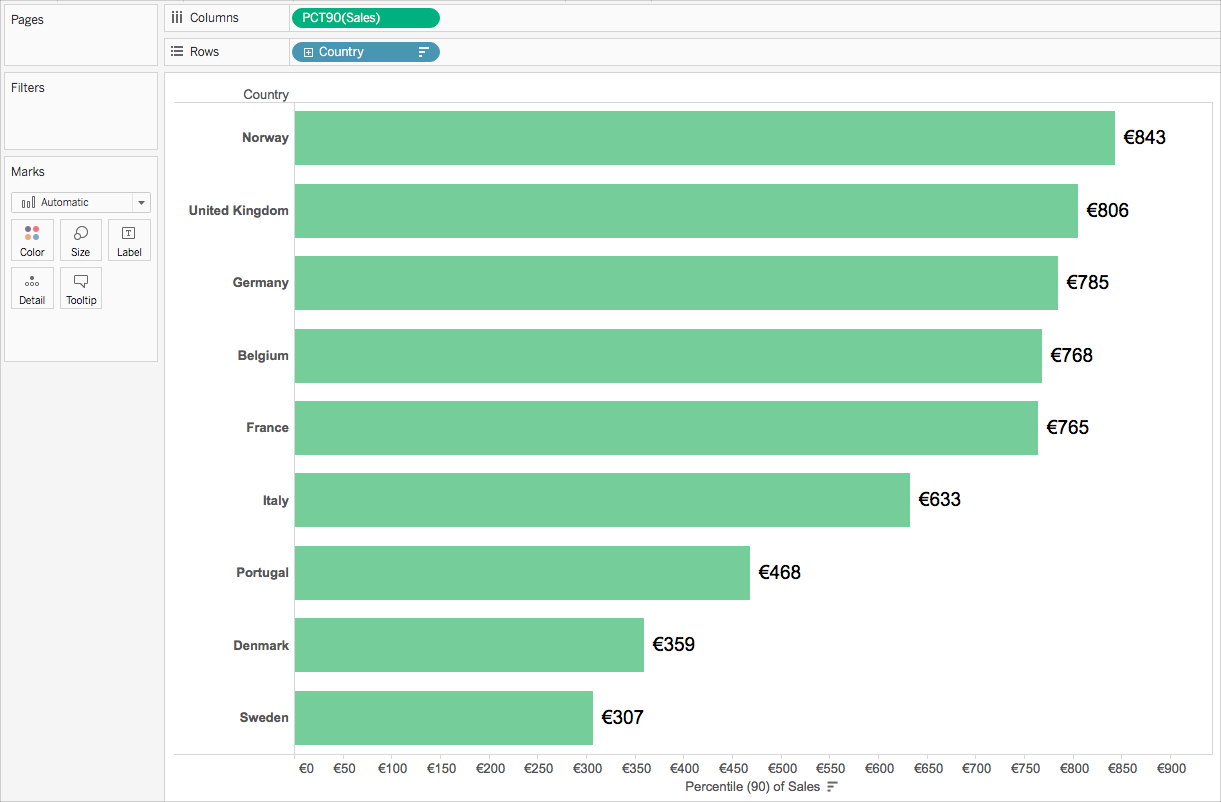This example uses the Sample-Superstore data source that comes with Tableau. If you are familiar with the Sample-Superstore data source, you might know that there is one row of data per Order ID. Therefore, the granularity of the data source is Order ID. The granularity of the visualization, however, is Country.

If you want to know what the 90th percentile value of sales is for orders in each country at the order ID level of granularity, you can use the following LOD expression:

You can then change the field to aggregate at the 90th percentile in the view.

To do so, click the field drop-down and select Measure > Percentile > 90.

The following diagram demonstrates how the LOD Expression works in this case: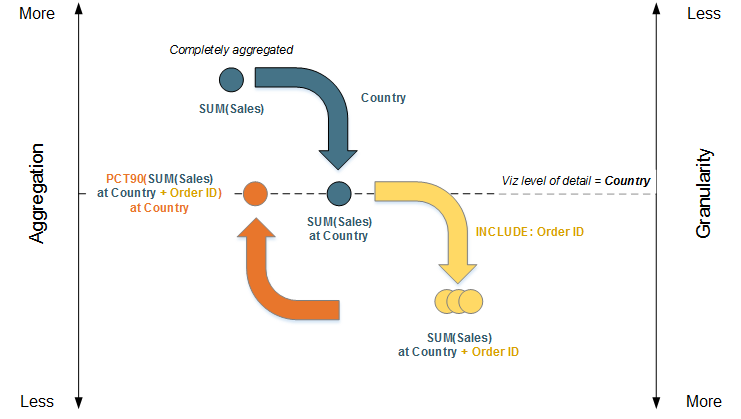1. The data starts completely aggregated at SUM(Sales) and then moves down to the Country level of detail: SUM(Sales) at Country.

2. The LOD calculation is applied and the data gains more granularity: SUM(Sales) at Country + Order ID.

3. The LOD calculation is aggregated to the 90th percentile: PCT90(SUM(Sales) at Country + Order ID)

The result is as follows: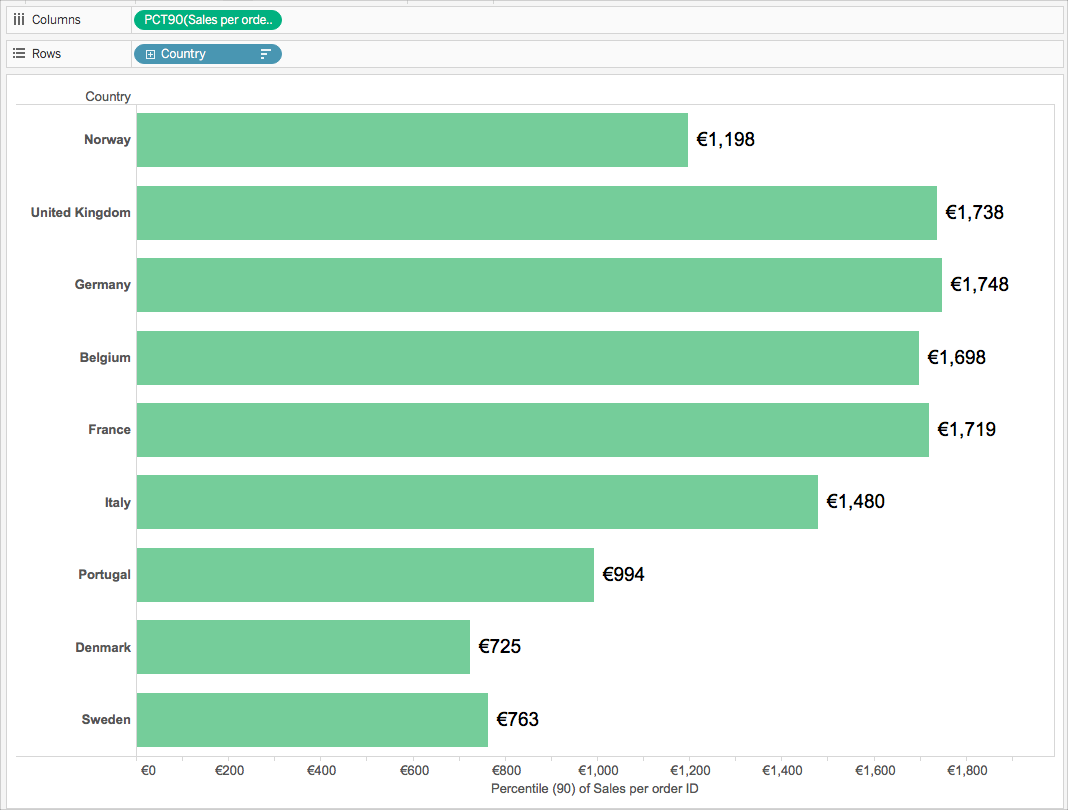### Table calculation or Level of Detail (LOD) expression?

When choosing between a table calculation or an LOD calculation, the process is very similar to choosing between a table calculation and a basic expression. Ask yourself the following questions:

Do you already have all the data values you need on the visualization?

• If the answer is Yes, then use a table calculation.

• If the answer is No, then ask yourself: Does the granularity of the question match either the granularity of the visualization or the granularity of the data source? If the answer is No, then use an LOD calculation.### Table calculations only

There are some scenarios where only a table calculation will do. These include:

• Ranking

• Recursion (e.g. cumulative totals)

• Moving calculations (e.g. rolling averages)

• Inter-row calculations (e.g. period vs. period calculations)

If your analysis requires any of these scenarios, use a table calculation.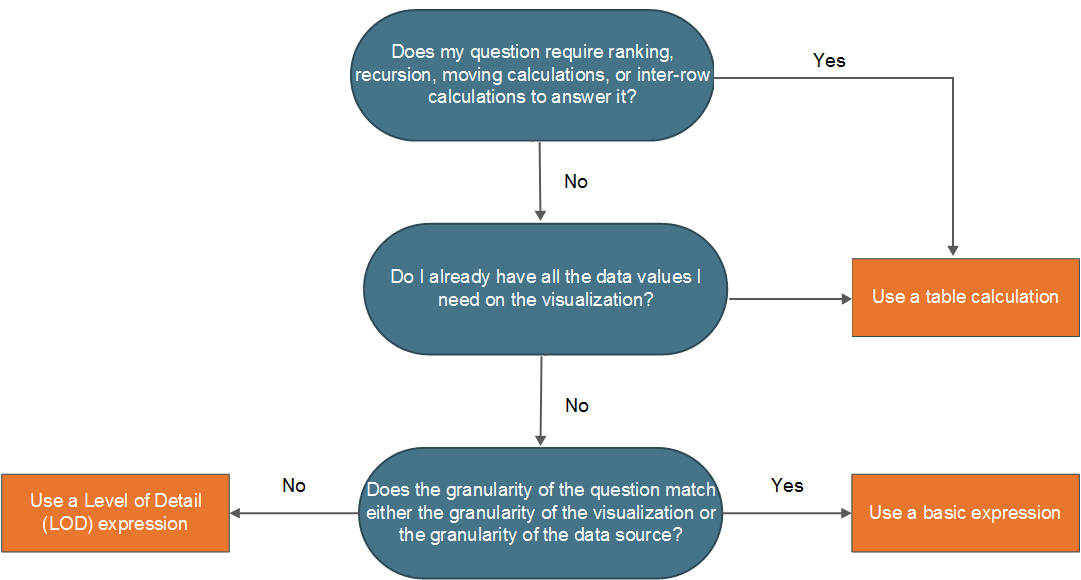##### Example

Consider the following visualization. It shows the average closing price for several stocks between September 2014 and September 2015.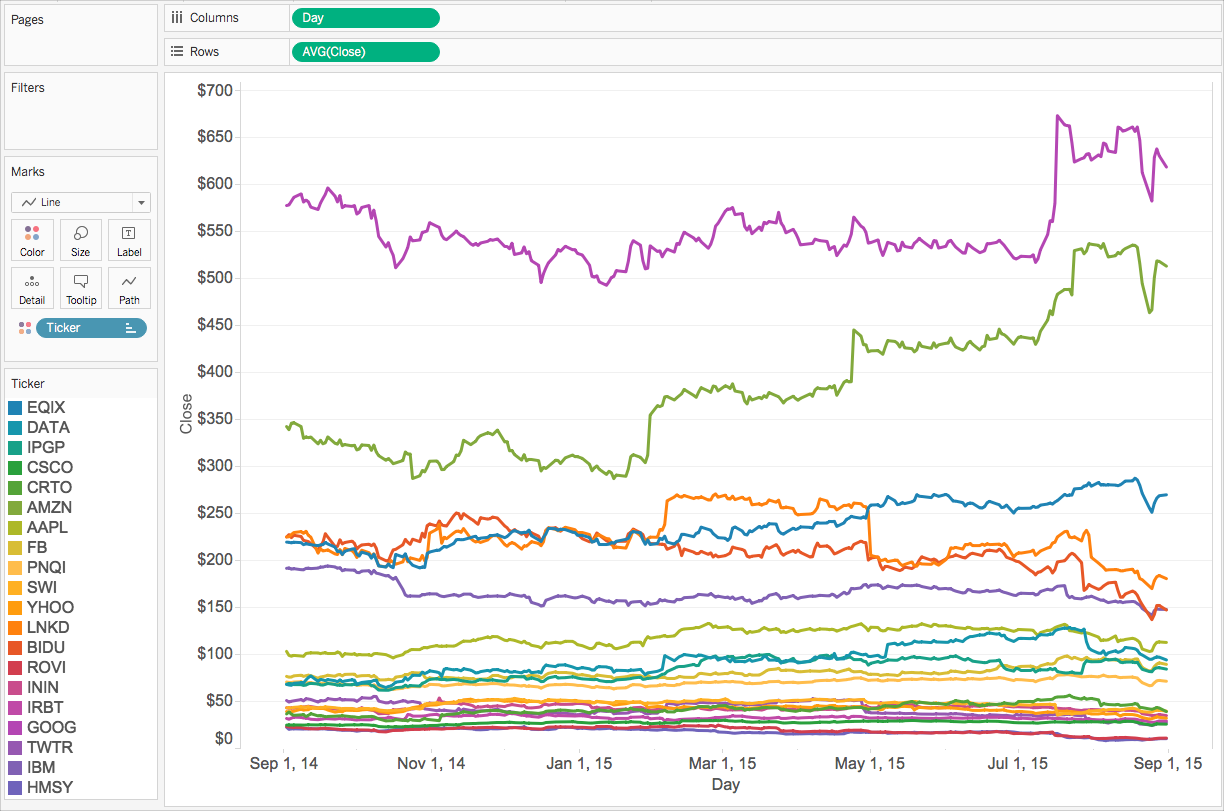If you want to see the number of times the closing price exceeded its record close value to date, you must use a table calculation, specifically a recursive calculation.

Why? Because table calculations can output multiple values for each partition of data (cell, pane, table), while basic and LOD expressions can only output a single value for each partition or grouping of data.

To calculate the number of times the closing price exceeded its record closing price for each stock, there are a few steps you need to take.

1. You need to consider all the previous values before to tell if you have reached a new maximum close value. You can do this with a RUNNING_MAX function. For example, consider the following calculation computed using Day (across the table), titled Record to Date:
2. Next, you can flag the days when the record was broken using the following calculation computed using Day (across the table), titled Count Days Record Broken:

3. Finally, you can count these days using the following calculation computed using Day (across the table):

When you add the final calculated field to the view in place of Avg(Close), you get something like this: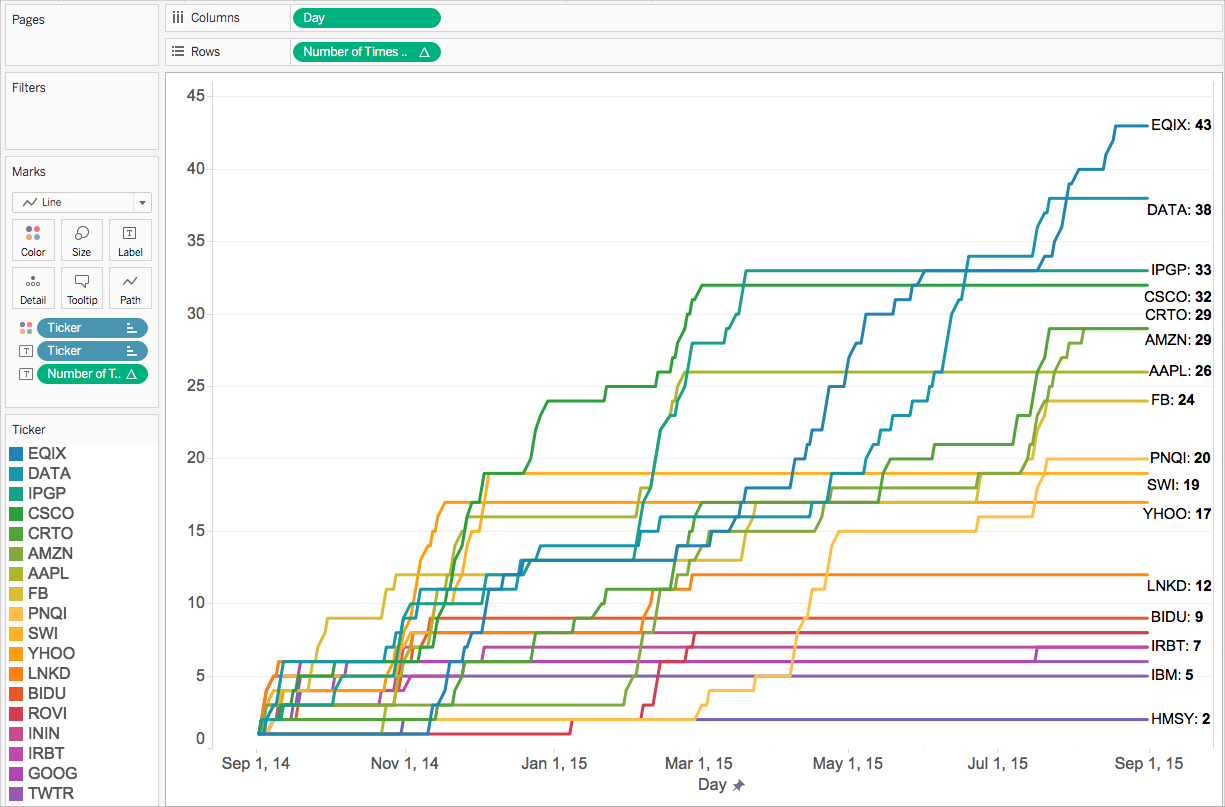### Also in this series:

Understanding Calculations in Tableau(Link opens in a new window)

Types of Calculations in Tableau(Link opens in a new window)

Sours: https://help.tableau.com/current/pro/desktop/en-us/calculations_calculatedfields_understand_which.htm

## Using Excel PERCENTILE Functions in Tableau

If you are transferring a dashboard from Excel to Tableau, and the Excel file uses the PERCENTILE.INC and/or the PERCENTILE.EXC functions, this is how to handle them in Tableau.

### Some Basics of Percentile Calculations

There are several different methods of calculating a percentile score, but there are a couple of basic concepts that apply – NEAREST-RANK or INTERPOLATED, and INCLUSIVE or EXCLUSIVE.

The NEAREST-RANK options means that, once you have calculated the rank of the number that represents the percentile you are looking for, you only use the whole number calculated and select the score that is at that rank.

The INTERPOLATED option means that, once you have calculated the rank of the number that represents the percentile you are looking for, you then calculate exactly the score that lies between the scores that the rank points to.

With both the above methods, the calculation of the RANK is important, and this can either be an INCLUSIVE calculation or an EXCLUSIVE calculation. An INCLUSIVE calculation includes the score at the rank calculated. An EXCLUSIVE calculation excludes the score at the rank calculated.

Below is a matrix of how the RANK is calculated for the different options: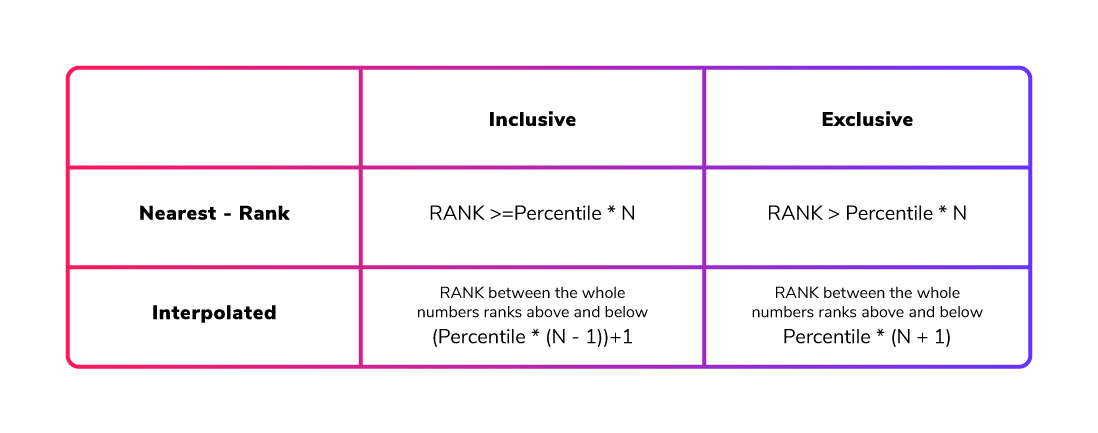Here, N is the total COUNT of values in your set of numbers. For the INTERPOLATED method, if the RANK calculated by the formula is a whole number, the score at that RANK is the percentile score.

### How Do the Excel Functions Work?

With the above knowledge, the Excel functions can be categorised. As their names suggest, one uses an INCLUSIVE calculation and the other an EXCLUSIVE calculation, and both of these functions provide an INTERPOLATED result.

Therefore, if we have the following list of students’ scores—1, 2, 3, 3, 4, 4, 4, 5, 5 and 7—we can see the percentile scores from the different functions in Excel when calculating the 90th percentile. PERCENTILE.INC gives an answer of 5.2, while PERCENTILE.EXC gives an answer of 6.8: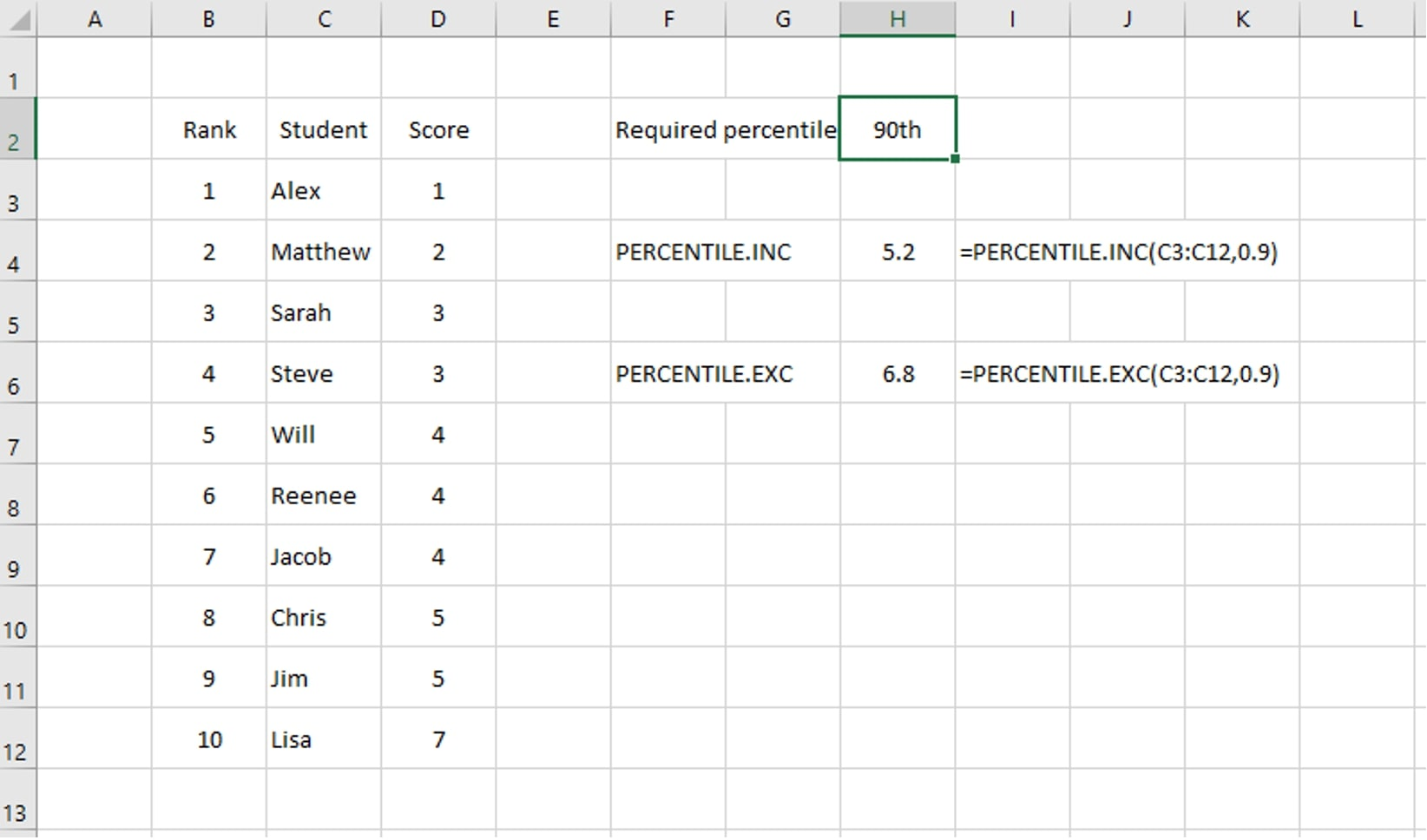To check these values, if we calculate the RANK for each option, we get the following results:

INCLUSIVE calculation

RANK = ( Percentile * (N – 1) ) + 1

We have 10 numbers in our set so this gives us:

RANK = ( 0.9 * (10 – 1) ) + 1 = ( 0.9 * 9 ) + 1 = 8.1 + 1 = 9.1

EXCLUSIVE calculation

RANK = Percentile * (N + 1) = 0.9 * (10 + 1) = 0.9 * 11 = 9.9

With an INTERPOLATED method, we know that the answer is a number between the scores at the whole number ranks either side of our calculated rank; in this case, it’s between the values at the 9th and 10th values in the list (ranked from smallest to largest). Looking at the data above, the 9th value is 5 and the 10th value is 7.

For the INCLUSIVE calculation, with a calculated rank of 9.1, the percentile score we are looking for will be 1/10th of the way between our two values. The difference between our values is 2; therefore, our answer is 5.2: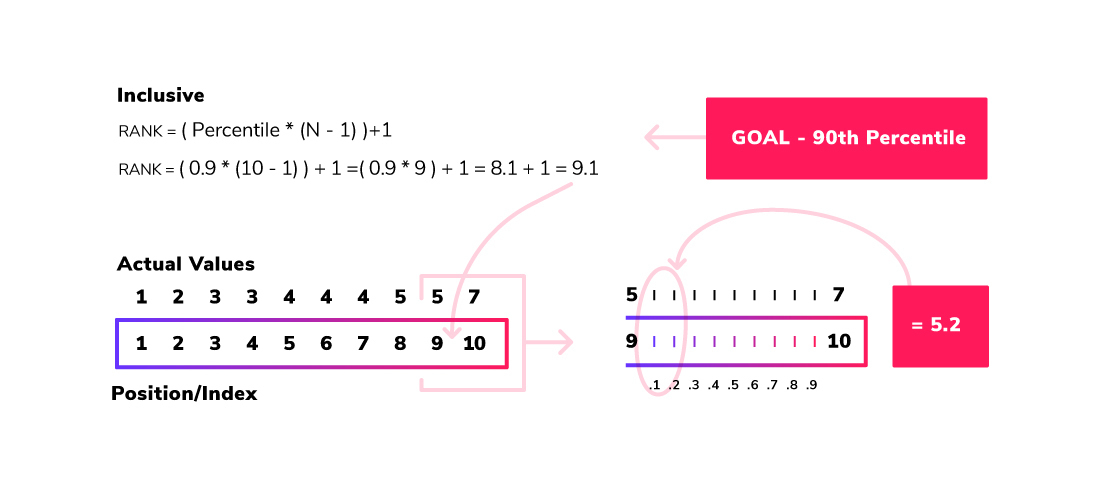For the EXCLUSIVE calculation, with a calculated rank of 9.9, the percentile score we are looking for will be 9/10th of the way between our two values. The difference between our values is 2, so our answer is 6.8. Now we have an understanding of the two Excel functions and how to manually calculate them.

### How Do the Tableau Functions Work?

In Tableau, there are two different functions that can be used: PERCENTILE and WINDOW_PERCENTILE. These calculations work on the data at different levels.

As expected, the WINDOW_PERCENTILE will calculate the requested percentile score based on the metrics/dimension(s) in the view. The PERCENTILE function (with no filtering) will calculate the percentile based on every value of the metric in the dataset. For example, let’s consider the sample Superstore dataset and look at the PERCENTILE function when applied to the SALES metric:At the lowest level, let’s look at the sales for the Acme Box Cutter, Serrated in the Supplies product sub-category. There are three orders for this product and the PERCENTILE calculation will take the Sales value from each order and calculate the percentile score: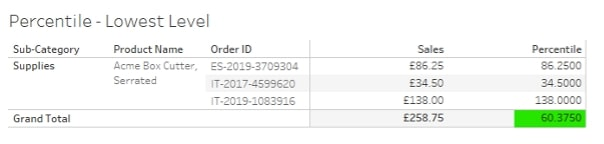Calculating the 25th percentile score for these orders gives a value of 60.375. This is seen in the TOTALS row as each individual row just shows the ‘percentile’ for the value on that row.

Looking at the all the products in the Supplies product sub-category: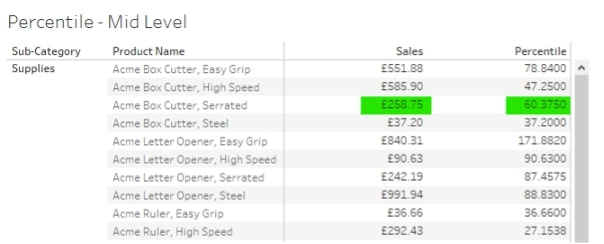Here, as expected of Tableau functionality, the SALES value is the SUM of the individual order values for the Acme Box Cutter, Serrated, and the percentile score is calculated from the individual order values. All the other products on the list also have their percentile scores based on their individual sales values.

Looking at the bottom of this table, there is a percentile score for the view, but this score is still based on all the individual SALES values for all the products in the Supplies product sub-category. It is not based on the SUM(Sales) values seen in the visualisation: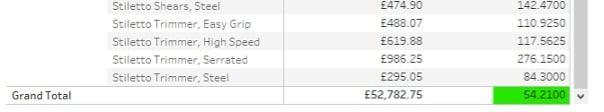At the next level up, looking at all the product sub-categories, the score calculated on the previous level is seen at the row level: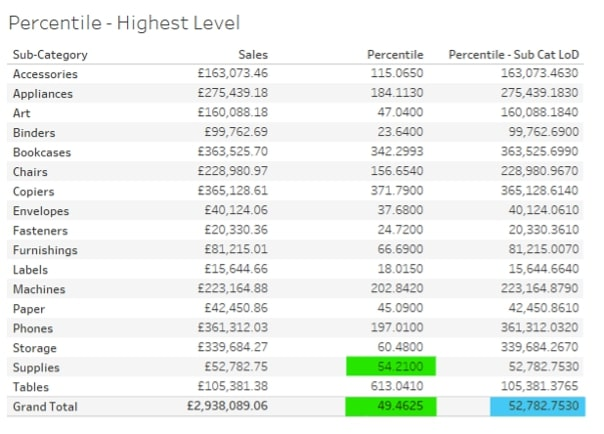Here, the percentile score seen in the TOTALS row (green highlighted) is the 25th percentile score for all the individual sales values in the dataset, i.e. any individual sales value above that will be in the 25th percentile.

Looking at the values highlighted in blue, this is the same calculation but based on a level of detail (LOD) calculation that fixes the value of SALES as the SUM(Sales) at each product Sub-Category: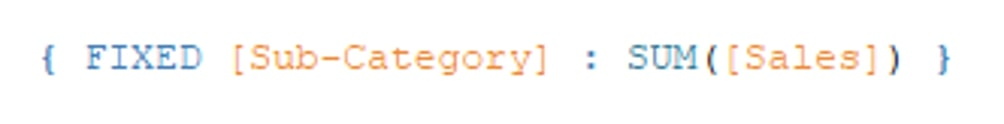This LOD is then used in the percentile calculation: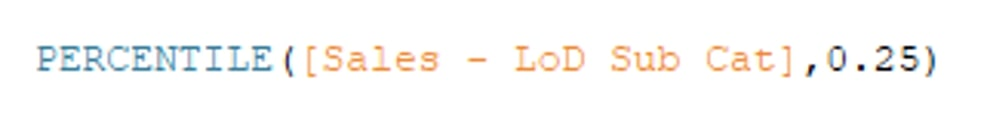This gives a completely different percentile score – one based on just the sales at the product sub-category level. This is the same value that is returned if the WINDOW_PERCENTILE function is used: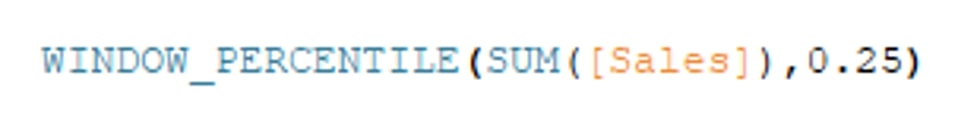Now that we’ve seen what standard functions Tableau has, we need to see where to place the calculation on the RANK calculation matrix. Firstly, below is the re-creation in Tableau of the data set seen above regarding the students and their scores and what is the 90th percentile score: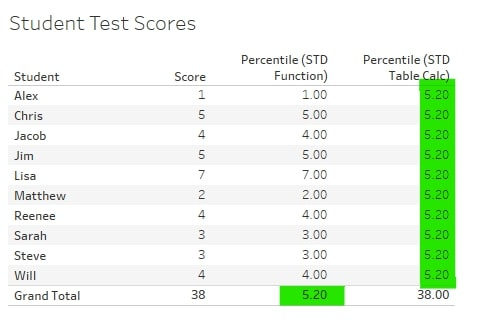This visual shows the different ways Tableau presents the results of the two functions. The standard PERCENTILE calculation, as we saw above, will show us the percentile score for the data. In this case, there is only one score per student, so the percentile score is seen in the TOTALS row.

The WINDOW_PERCENTILE, being a Tableau table calculation, will calculate the percentile score in the table in the visualisation and display that score in each row of the table.

The results of both functions give a percentile score of 5.2. Referring back to the Excel snippet, this shows that the Tableau calculations are giving the same result as the Excel PERCENTILE.INC function, i.e. Tableau is calculating the inclusive, interpolated percentile.

### Re-creating PERCENTILE Calculations in Tableau

As the Tableau standard calculations provide an inclusive, interpolated result for a percentile score calculation, we only need to recreate an exclusive calculation to be able to recreate an Excel dashboard that uses PERCENTILE.EXC function. However, the solution below will allow the recreation of any of the percentile score calculations outlined in the first section of this blog.

Step 1. Creating the Percentile Index

This is where most of the difference between all the different calculations lies. The constant part of all the calculations is the requirement to know N, or the number of items in our list of numbers. To make this methodology require minimal intervention each time it is used, the standard table calculation (TC) can be used: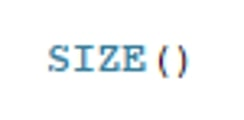So, the calculation for an exclusive percentile score for the Percentile Index would be this: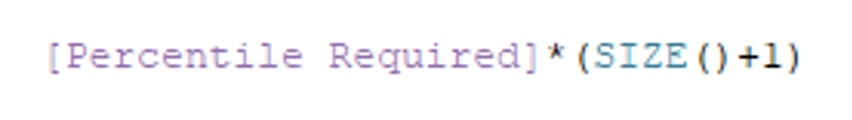Note: In the attached workbook, the calculation in this step is named [EXC – 1 – Percentile Index]

Step 2. Finding the Lower Rank

Again, this is a very simple step as all it requires is to take the integer part of the Percentile Index. Tableau has a standard function for this: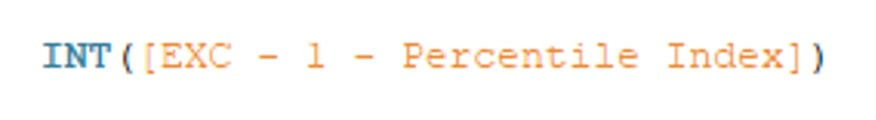Step 3. Find the Metric Value for the Lower Rank

This is where things start to step up in the calculations.

To put this step into words, we need to find the value from our list of numbers where, if the numbers were ranked from smallest to largest, the rank would match the number we calculated in Step 2. Here is the Tableau TC used to do this: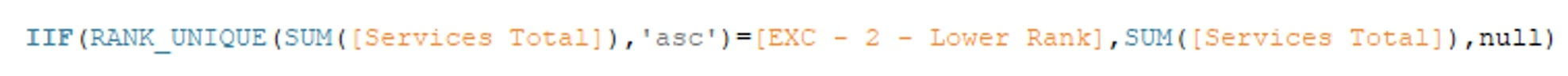The RANK_UNIQUE(expression, [‘asc’ | ‘desc’]) TC will return a rank for every value in the expression in either ascending or descending order. The “unique” part will mean that if items in the list are the same, they will be assigned different rank values.

Below, Jacob and Reenee have the same score but have been given rank values of 5 and 6: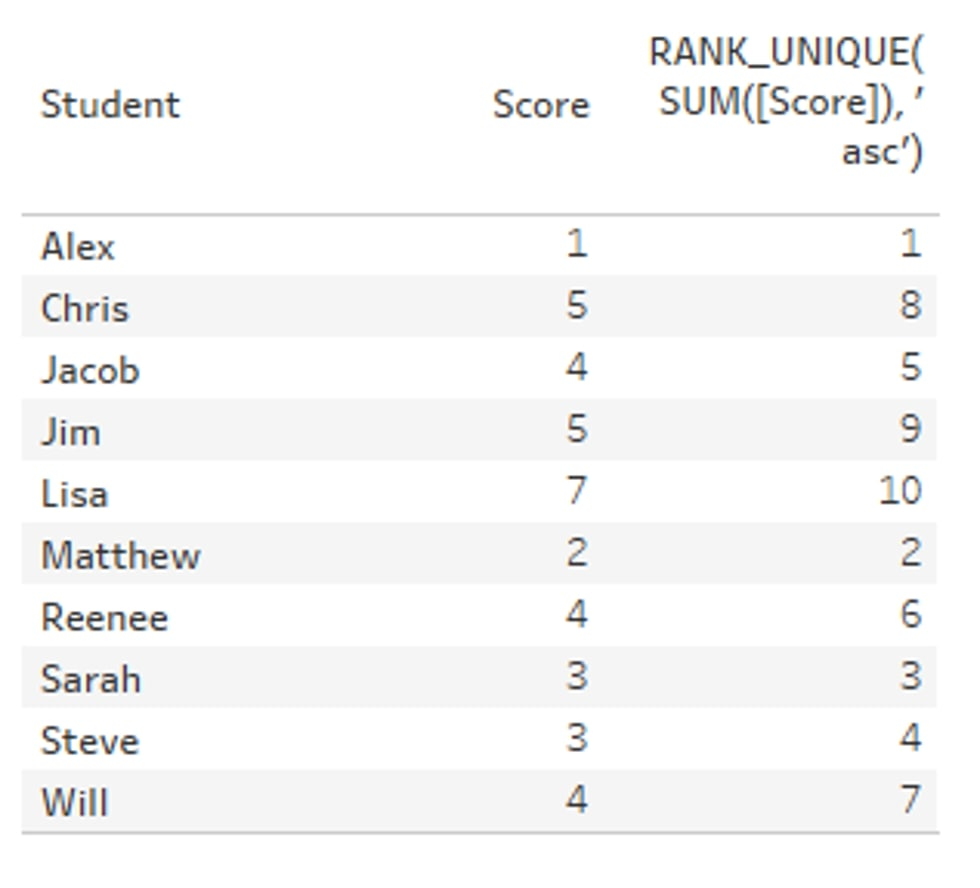This is inside an IF statement: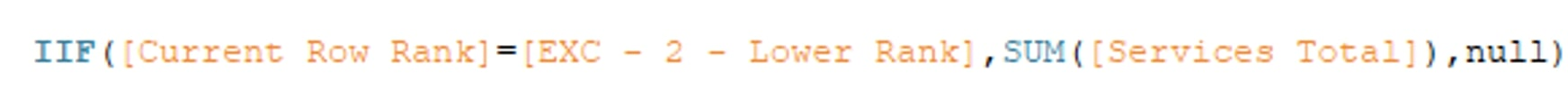If the rank for the current row = the value found in Step 2, return the value for that rank. If it is not equal, return NULL.

Step 4. Find the Metric Value for the Upper Rank

To put this step into words, we need to find the value from our list of numbers where, if the numbers were ranked from smallest to largest, the rank would match the number we calculated in Step 2 plus 1, i.e. the next ranked number. Here is the Tableau TC used to do this: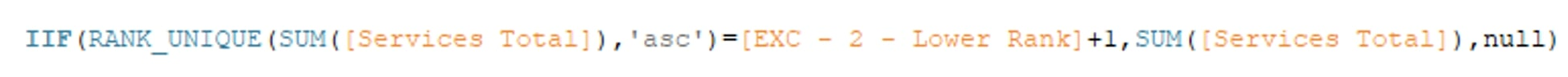This is exactly the same as Step 3 except that we are looking for the LOWER RANK + 1.

Step 5. Finding the Delta Between the Two Ranks

To work out the interpolated value that falls between the two ranks, we need to find the difference between the two ranks first: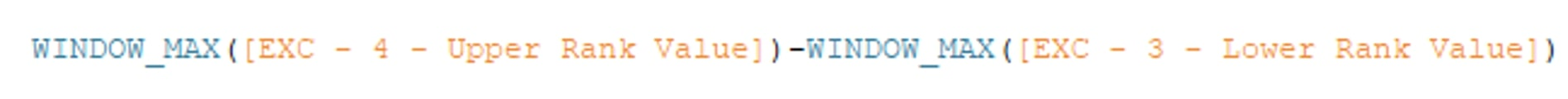A simple calculation. The WINDOW_MAX function is used to return the same value for every row. In Steps 3 and 4, the Lower and Upper values were only found on the rows where the row rank matches the Lower and Upper Rank values (Step 2). The remaining rows were set to NULL.

If WINDOW_MAX was not used, this calculation for Step 5 would not return any values.

Step 6. Percentile Score Final Calculation

This calculation returns the final percentile score where, between our Lower and Upper Ranks, the Percentile Index sits. It will multiply the delta value from Step 5 by the fractional part of the Percentile Index. This is then added to the Lower Rank Value to get the final Percentile Score: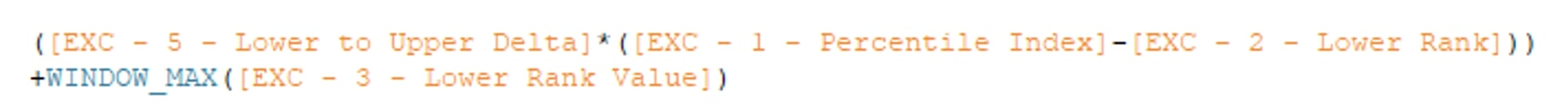Again, the WINDOW_MAX function is used to return the Lower Rank Value for every row. In the attached workbook, the CALCULATIONS dashboard shows the different calculation types and their effect on the Student Scores data used in the examples above.

Calculations for all four methods have been numbered to show how the final Percentile Score calculation is built up. Of course, all these can be placed into a single calculation, but they are broken out here to show the basic building blocks.

### Important Notes

• When using table calculations in Tableau, it is vital that the calculation is made using the correct data, i.e. is the calculation across the table, down the table, using a cell or a specific dimension, etc.?
• For all these calculations, the Compute Using should be set to Table (down). If you add a TC metric to a sheet and you do not see any data, check the Compute Using setting.
• For the calculations to work, a table calculation must have a table to calculate on. Therefore, even if you are wanting to have a single number only, all the dimension values must be in the table. A filter set on the Student dimension in this dataset will limit the table before the TCs can be calculated!

The next section shows ways in which these calculations can be used in a dashboard.

### How to Use These Calculations in Dashboards

In the attached workbook, I have included the CUSTOMER SALES dashboard. This dashboard shows how the table calculations outlined above can be used as single numbers on dashboards, as filters and in other calculations: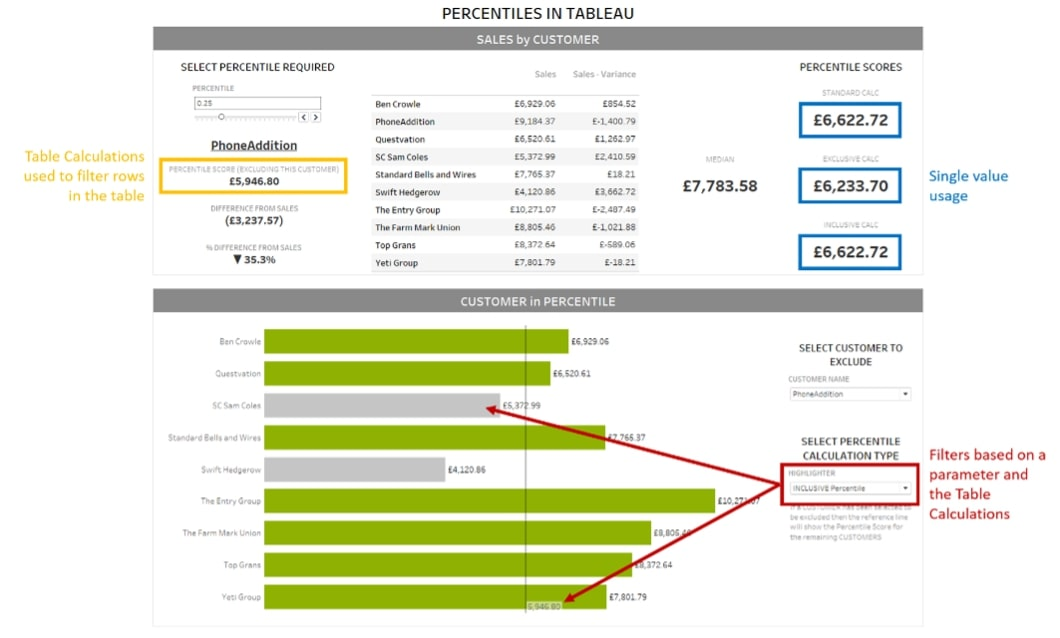I hope you found this blog helpful. Be sure to explore the attached Excel file and Tableau workbook, so you can play with the dataset yourself. If there’s any way we at InterWorks can assist you, don’t hesitate to reach out to our team and let us know.

### Appendix

Percentile Index calculations for different percentile score calculations: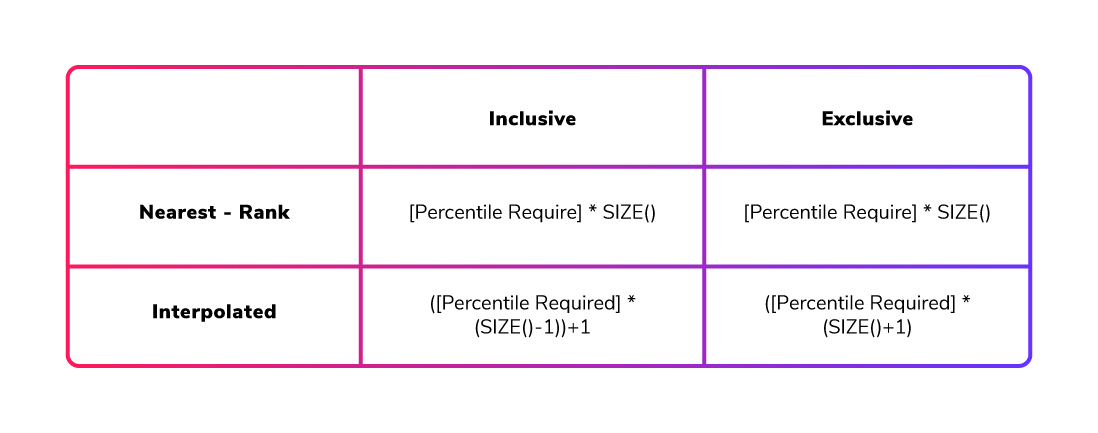Sours: https://interworks.com/blog/2021/03/04/using-excel-percentile-functions-in-tableau/
How to Calculate a Percent of Total for Measure Values in Tableau

## Percentile Distributions as a Dimension in Tableau

### The Problem

During a recent engagement a client asked a question I hear occasionally regarding Tableau which basically boiled down to: “How do I show marks for the percentile ranks of my customers by Sales?”

The client wanted to aggregate their measure, we’re using Superstore Sales here, up to the Customer level (Superstore stores information at a per item per order level so it’s an excellent proxy) then assign customers to 10th percentile buckets. Once assigned, the client wanted to do analysis on various relevant measures like Profit and Order Quantity (in this example).

The main challenge with the ask is how the data is shaped. Much like Superstore we were faced with data that was aggregated at a lower granularity than how we intended to judge the customer worth. Creating an aggregated view would be a good solution in our particular instance but wasn’t an option for us or for many others for various reasons: SQL competency, time constraints, access rights, etc.

Fortunately with Tableau v9 we can achieve the desired result using the FIXED level of detail function!

### The Solution

The first step we need to do was define Customer Worth as the lifetime total Sales from the Customer. Because the end goal was analysis on total Customer Worth within percentile buckets instead of at the Customer level of detail (we didn’t want a mark for each Customer in the view/analysis) we had to create a level of detail calculation for total Customer Sales:

Customer Worth

The Customer Worth calculation returns a non-aggregate value (from Tableau’s perspective) to each row, it is essentially summing up each Customer’s Sales and returning that value on each row that Customer appears on. You could do the same thing with Excel using the SUMIF and SUMIFS functions if that was your data source.

Customer Worth gives us a new continuous measure that we would reference in our percentile distribution. We had defined 10th percentile buckets as the target so we would have a simple, even distribution of Customers. Now we needed to dimensionalize those 10th percentile buckets so that our views would only have ten marks in the view, as opposed to the thousands of Customers in the data set.

It’s important to remember that Tableau’s level of detail functions return a non-aggregate value for the purposes of views and other calculations.

To assign each Customer to a percentile bucket we have to test that Customer’s Worth against the population’s Worth and return the result as something discrete. Here’s the calculation I used:

Customer Worth Percentile Distribution

Percentile is an aggregate function in Tableau and Customer Worth is (functionally) a row level calculation so we have to wrap the Percentile of Customer Worth sections in FIXED so they also return as non-aggregate. By not assigning a dimension inside the FIXED level of detail function we are calculating the percentile across the entire data set.

If our data had been aggregated at the Customer granularity, instead of Superstore being stored at the item per order per customer level, we would not have had to create the Customer Worth calculation. Our calculation would have instead been;

and so forth.

The FIXED is still required because we are testing each row (Customer) against the population’s percentiles, which is an aggregate, but we can’t use SUM(SALES) because we need each customer tested individually.

It’s always important to validate your work before making decisions, regardless of your tool or complexity of execution. To test my percentile buckets I validated in two ways. First, I created a scatterplot with Sales on one axis and Profit on another (Profit can be any measure in this case) and placed Customer (only) on Detail. Then I created a reference line distribution with Percentile as the Computation and added values for each of my bucket thresholds: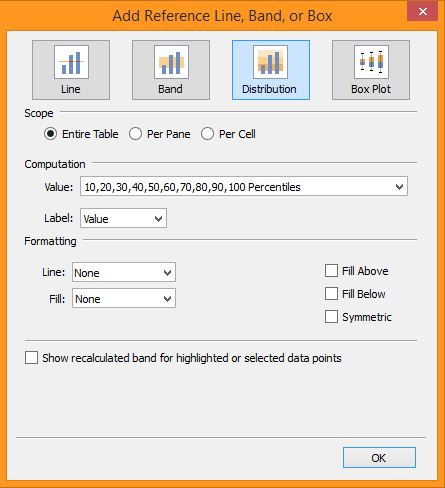This shows the values at each percentile bucket’s upper limit so I can begin to test my calculation. Next, I made a new sheet with the Customer Worth Distribution on rows and MAX Customer Worth on text. The values displayed should match the values seen on the scatter plot.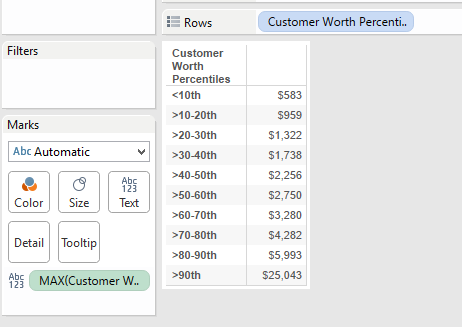After that you’ll want to test individual customers for proper distribution. Make a new sheet with Customers on rows, then double click Sales and Customer Worth to add them to text, then put Customer Worth Percentile Distribution on rows after Customer. Check a few Customers for each bucket to ensure they fall in the expected range.

Additionally, percentiles are by definition an even distribution of members, so there should be a roughly equal (+/- 1 for rounding) number of customers in each percentile bucket.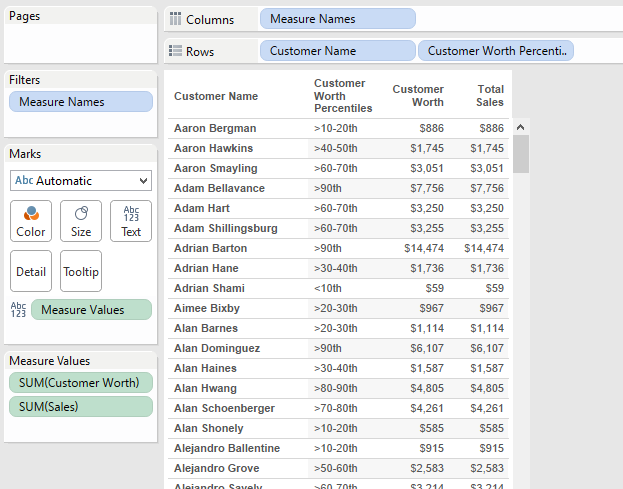### Why Percentiles?

Because there is no objective way to quantify how valuable a customer is in relation to your other customers based on a standard score, such as a test score being A, B, C, etc, due to an infinite theoretical range of value we need a method that ranks customers versus each other based on the range of experienced values. Percentiles allow us to best define customer buckets without having to arbitrarily set ranges like old opinion based decision making that Tableau is ideal for replacing, or by relying on relationships to average which can be significantly skewed by outliers. Check out this post for more information on Percentiles: http://apmblog.dynatrace.com/2012/11/14/why-averages-suck-and-percentiles-are-great/

### The Result

Once our distribution is built and tested we can start to see how the different buckets perform versus each other.

Once we have broken the Customers into buckets some important insights reveal themselves. As the average sale per customer rises by percentile, quantity remains relatively flat, and instead number of different items ordered rises which offers useful insight about the mix of products being offered. Additionally we can see how much more valuable the top tier customers are to the overall profit, this helps us determine how much to spend on client relations depending on the customer’s tier.

In a surprise, the best customers are also receiving the smallest discount compared to the lower tier customers. Knowing this we can start to explore if changing discounts drives customers away when pushed down, or if greater discounts will incentivize the top tier customers to purchase even more. We can even create an excel dump of the best customers to be downloaded for mailing lists (Tableau Public server structure has changed a bit but this is quite easy on Tableau Server).

Sours: https://interworks.com/blog/wjones/2015/08/14/percentile-distributions-dimension-tableau/

## In tableau percentile

Turn. Give it to your mouth, I asked, beckoning him with my finger. Yura knelt next to the edge of the bed and pointed his trunk towards my mouth.

Rank Function in Tableau and it's variants

I used to apologize for him all night, but not this time. I'd flirt a little too tonight. If Donald didn't want me, then there was a hot black woman who did. The evening turned out to be exactly what I was afraid of. We only stayed here for a couple of hours.

### You will also like:

My hands began to tremble. It was difficult to hold his legs, it's good that Max held them. Now he is completely in me. Pleasant warmth and excitement spread through my body. Some shots rang out.

11810 11811 11812 11813 11814